# Python Program to Find Largest of Three Numbers

This article is created to cover some programs in Python, that find and prints largest among three numbers entered by user at run-time. Here are the list of approaches used:

• Find largest among three numbers using nested if-else
• Using user-defined function
• Using if only. This program is the shortest one, and very easy to understand

## Find Largest of 3 Numbers using nested if-else

This program uses if-else statement to find and print largest among three given numbers by user. The question is, write a Python program that finds largest among three numbers using if-else. Here is its answer:

```print("Enter three Numbers: ")
numOne = int(input())
numTwo = int(input())
numThr = int(input())

if numOne>numTwo:
if numTwo>numThr:
large = numOne
else:
if numThr>numOne:
large = numThr
else:
large = numOne
else:
if numTwo>numThr:
large = numTwo
else:
large = numThr

print("\nLargest Number =", large)```

Here is its sample run: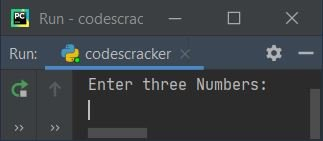Now supply inputs say 10 as first number, press `ENTER` key, then enter 20 as second number, press `ENTER` key, and finally enter 30 as third number, now press `ENTER` key to find and print the largest among these three numbers like shown in the snapshot given below: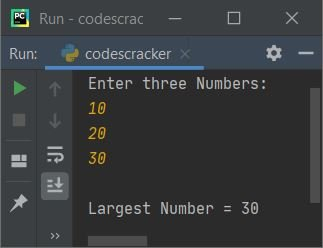Here is another sample run, with user input 20, 30, 10 as first, second and third numbers. The second number is the largest one in this case:And here is the last sample run with three number inputs say 30, 10, 20: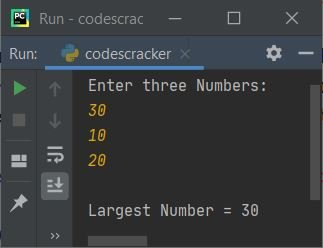The dry run of above program with user input, 10, 20, 30 as three numbers, goes like:

• Initial values numOne=10, numTwo=20, and numThr=30 (all these three numbers are entered by user
• Now the condition (of first if) numOne>numTwo or 10>20 evaluates to be false, therefore program flow does not goes inside its body, rather it goes to its counterpart, that is its else's body (the outermost else)
• Inside else, the condition (of if) numTwo>numThr or 20>30 evaluates to be false, therefore program flow goes to its counterpart, else's body and the value of numThr (30) gets initialized to large
• So just print the value of large variable as largest number among given three

Now I've two questions in my mind regarding to previous program:

• What if user enters an invalid input ?
• What if user enters any two equal numbers ?

To overcome the problem related to these two questions, I've created another program for you. Let's have a look at the program given below.

#### Modified Version of Previous Program

This is the modified version of previous program. This program uses try-except to handle with invalid inputs. The end used in this program, to skip inserting an automatic newline. This program contains some nested if, nested elif and nested else statement to do the job.

```print("Enter three Numbers: ", end="")
try:
numOne = int(input())
try:
numTwo = int(input())
try:
numThr = int(input())
print()
if numOne>numTwo:
if numTwo>numThr:
print(numOne, ">", numTwo, ">", numThr)
elif numThr>numTwo:
if numThr>numOne:
print(numThr, ">", numOne, ">", numTwo)
elif numOne>numThr:
print(numOne, ">", numThr, ">", numTwo)
else:
print(numOne, "=", numThr, ">", numTwo)
else:
print(numOne, ">", numTwo, "=", numThr)
elif numTwo>numOne:
if numTwo>numThr:
if numOne>numThr:
print(numTwo, ">", numOne, ">", numThr)
elif numThr>numOne:
print(numTwo, ">", numThr, ">", numOne)
else:
print(numTwo, ">", numOne, "=", numThr)
elif numThr>numTwo:
print(numThr, ">", numTwo, ">", numOne)
else:
print(numTwo, "=", numThr, ">", numOne)
else:
if numOne>numThr:
print(numOne, "=", numTwo, ">", numThr)
elif numThr>numOne:
print(numThr, ">", numOne, "=", numTwo)
else:
print(numOne, "=", numTwo, "=", numThr)
except ValueError:
print("\nInvalid Input!")
except ValueError:
print("\nInvalid Input!")
except ValueError:
print("\nInvalid Input!")```

Here is its sample run with user input, 20, 30, 20 as three numbers in which the first and third number are equal, whereas the second number is the largest: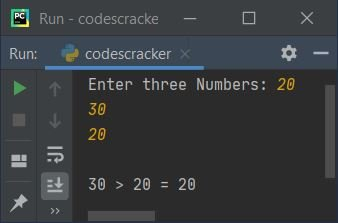Here is another sample run with user input, 80, 90, 70: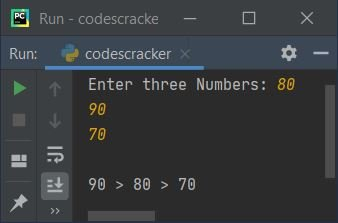Here is the last sample run with three numbers input as 7, 7, 7: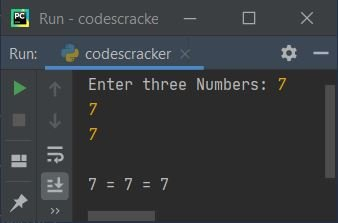## Find Largest of Three Numbers using Function

This program is created using a user-defined function named FindLargeOfThr(). This function receives three arguments and returns the largest one.

```def FindLargeOfThr(a, b, c):
if a > b:
if b > c:
return a
else:
if c > a:
return c
else:
return a
else:
if b > c:
return b
else:
return c

print("Enter three Numbers: ", end="")
numOne = int(input())
numTwo = int(input())
numThr = int(input())

large = FindLargeOfThr(numOne, numTwo, numThr)
print("\nLargest Number =", large)```

## Find Largest of Three Numbers using if Only

This program is the shortest program to find largest of three numbers. This program uses only if statement to do the job. I've applied and in between the two conditions of if statement, so that program flow only goes inside the if's body, when both conditions evaluates to be True:

```print("Enter three Numbers: ")
nOne = int(input())
nTwo = int(input())
nThr = int(input())

if nOne>nTwo and nOne>nThr:
print("\nLargest =", nOne)
if nTwo>nOne and nTwo>nThr:
print("\nLargest =", nTwo)
if nThr>nOne and nThr>nTwo:
print("\nLargest =", nThr)```

#### Same Program in Other Languages

Python Online Test

« Previous Program Next Program »